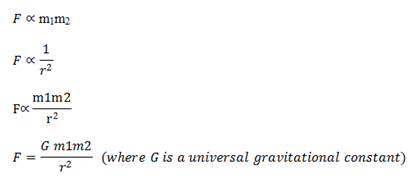# Gravitation

Newton’s law of gravitation
It states that everybody in this universe attracts other body with a force which is proportional to the product of the masses of two bodies and inversely proportional to the square of the distance between their centers.
From Newton’s law of Gravitation,If two masses of unit mass are placed at a unit distance apart then, F=G
So, G is numerically equal to the force between two bodies of unit masses kept unit distance apart. Its value is 6.67×10-11Nm2Kg-2. Its diameter is [L3M-1T-2].

Properties of G

It is independent of nature of masses
It is independent of medium in which the two masses are kept
Its value is same for small or large masses i.e. stars and galaxies to atoms

Properties of gravitational forces:
It is independent of the intervening medium between two bodies. It obeys Newton’s 3rd Law i.e. if body A exerts some force on body B then B exerts an equal but opposite force on A. So the force along the line joining the centre of two bodies is central and conservative in nature.

Gravitation & gravity
The force of attraction between any two large or small bodies is called gravitation. But if one of these forces happens to be earth then the force of attraction given by the earth on this small body is called gravity.